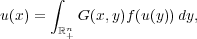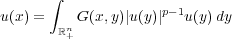#### Vol. 253, No. 2, 2011

 Download this articleFor screen For printingRecent Issues Vol. 317: 1 Vol. 316: 1  2 Vol. 315: 1  2 Vol. 314: 1  2 Vol. 313: 1  2 Vol. 312: 1  2 Vol. 311: 1  2 Vol. 310: 1  2Online Archive Volume: Issue:The Journal Subscriptions Editorial Board Officers Contacts Submission Guidelines Submission Form Policies for Authors ISSN: 1945-5844 (e-only) ISSN: 0030-8730 (print) Special Issues Author Index To Appear Other MSP Journals
Axial symmetry and regularity of solutions to an integral equation in a half-space

### Guozhen Lu and Jiuyi Zhu

Vol. 253 (2011), No. 2, 455–473
##### Abstract

We consider the integral equationwhere G(x,y) is the Green’s function of the corresponding polyharmonic Dirichlet problem in a half-space. We prove by the method of moving planes in integral form that, under some integrability conditions, the solutions are axially symmetric with respect to some line parallel to the xn-axis and nondecreasing in the xn direction, which further implies the nonexistence of solutions. We also show similar results for a class of systems of integral equations. This appears to be the first paper in which the moving plane method in integral form is employed in a half-space to derive axial symmetry.

We also obtain the regularity of the integral equation in a half-spaceby the regularity lifting method. As a corollary, we prove the nonexistence of nonnegative solutions to this equation. Moreover, we show that the nonnegative solutions in this equation only depend on xn if u Lloc2n∕(n2m)(+n) and 1 < p < (n + 2m)(n 2m).

##### Keywords
axial symmetry, regularity of solutions, half-space, Green’s functions for polyharmonic operators, integral equation, nonexistence of solutions
Primary: 35J60
Secondary: 45G15
##### Milestones